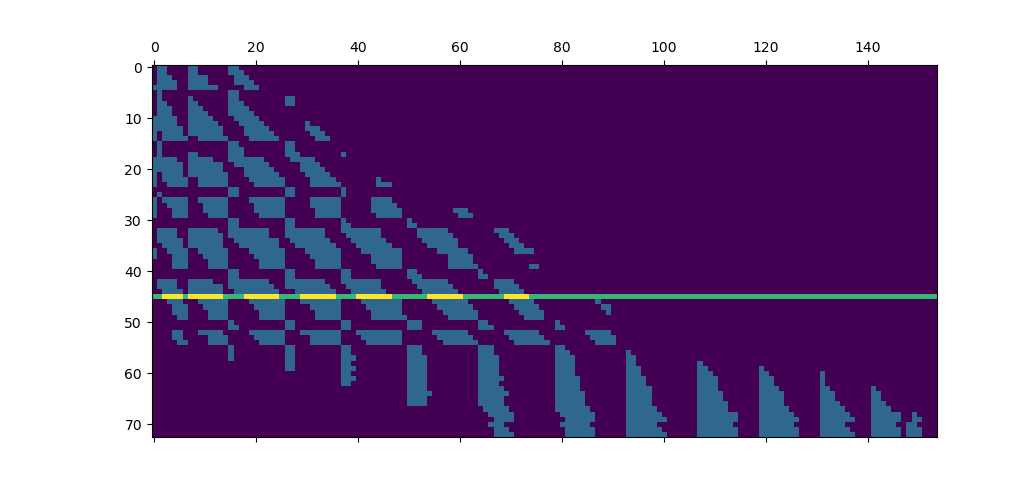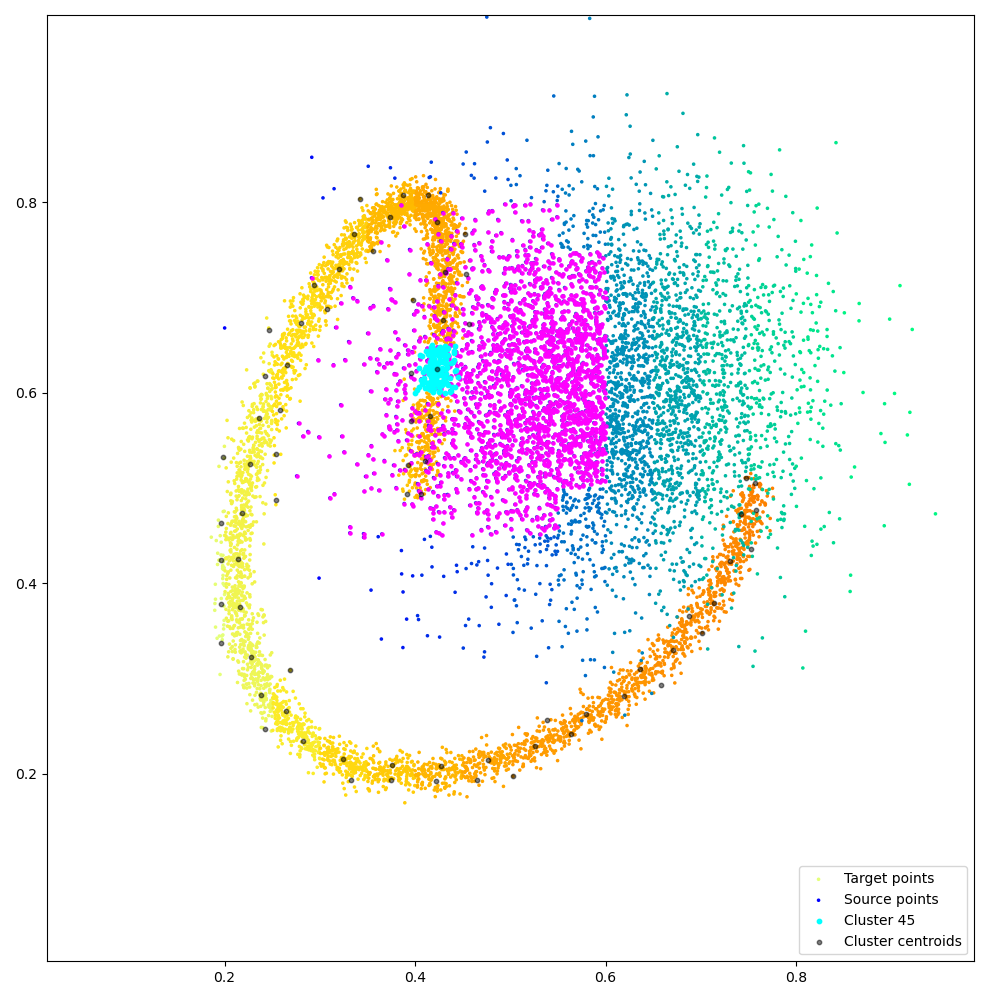# Block-sparse reductions

This script showcases the use of the optional ranges argument to compute block-sparse reductions with sub-quadratic time complexity.

## Setup

Standard imports:

import time

import numpy as np
from matplotlib import pyplot as plt

from pykeops.numpy import LazyTensor
import pykeops.config

dtype = "float32"


Define our dataset: two point clouds on the unit square.

M, N = (5000, 5000) if pykeops.config.gpu_available else (2000, 2000)

t = np.linspace(0, 2 * np.pi, M + 1)[:-1]
x = np.stack((0.4 + 0.4 * (t / 7) * np.cos(t), 0.5 + 0.3 * np.sin(t)), 1)
x = x + 0.01 * np.random.randn(*x.shape)
x = x.astype(dtype)

y = np.random.randn(N, 2).astype(dtype)
y = y / 10 + np.array([0.6, 0.6]).astype(dtype)


## Computing a block-sparse reduction

On the GPU, contiguous memory accesses are key to high performances. To enable the implementation of algorithms with sub-quadratic time complexity under this constraint, KeOps provides access to block-sparse reduction routines through the optional ranges argument, which is supported by numpy.Genred and all its children.

### Pre-processing

To leverage this feature through the pykeops.torch API, the first step is to clusterize your data into groups which should neither be too small (performances on clusters with less than ~200 points each are suboptimal) nor too many (the from_matrix() pre-processor can become a bottleneck when working with >2,000 clusters per point cloud).

In this tutorial, we use the grid_cluster() routine which simply groups points into cubic bins of arbitrary size:

from pykeops.numpy.cluster import grid_cluster

eps = 0.05  # Size of our square bins

Start = time.time()
start = time.time()
x_labels = grid_cluster(x, eps)  # class labels
y_labels = grid_cluster(y, eps)  # class labels
end = time.time()
print("Perform clustering       : {:.4f}s".format(end - start))


Out:

Perform clustering       : 0.0006s


Once (integer) cluster labels have been computed, we can compute the centroids and memory footprint of each class:

from pykeops.numpy.cluster import cluster_ranges_centroids

# Compute one range and centroid per class:
start = time.time()
x_ranges, x_centroids, _ = cluster_ranges_centroids(x, x_labels)
y_ranges, y_centroids, _ = cluster_ranges_centroids(y, y_labels)
end = time.time()
print("Compute ranges+centroids : {:.4f}s".format(end - start))


Out:

Compute ranges+centroids : 0.0002s


Finally, we can sort our points according to their labels, making sure that all clusters are stored contiguously in memory:

from pykeops.numpy.cluster import sort_clusters

start = time.time()
x, x_labels = sort_clusters(x, x_labels)
y, y_labels = sort_clusters(y, y_labels)
end = time.time()
print("Sort the points          : {:.4f}s".format(end - start))


Out:

Sort the points          : 0.0004s


The key idea behind KeOps’s block-sparsity mode is that as soon as data points are sorted, we can manage the reduction scheme through a small, coarse boolean mask whose values encode whether or not we should perform computations at a finer scale.

In this example, we compute a simple Gaussian convolution of radius $$\sigma$$ and decide to skip points-to-points interactions between blocks whose centroids are further apart than $$4\sigma$$, as $$\exp(- (4\sigma)^2 / 2\sigma^2 ) = e^{-8} \ll 1$$, with 99% of the mass of a Gaussian kernel located in the $$3\sigma$$ range.

sigma = 0.05  # Characteristic length of interaction
start = time.time()

# Compute a coarse Boolean mask:
D = np.sum((x_centroids[:, None, :] - y_centroids[None, :, :]) ** 2, 2)
keep = D < (4 * sigma) ** 2


To turn this mask into a set of integer Tensors which is more palatable to KeOps’s low-level CUDA API, we then use the from_matrix routine…

from pykeops.numpy.cluster import from_matrix

ranges_ij = from_matrix(x_ranges, y_ranges, keep)

end = time.time()
print("Process the ranges       : {:.4f}s".format(end - start))

End = time.time()
t_cluster = End - Start
print("Total time (synchronized): {:.4f}s".format(End - Start))
print("")


Out:

Process the ranges       : 0.0008s
Total time (synchronized): 0.0032s


And we’re done: here is the ranges argument that can be fed to the KeOps reduction routines! For large point clouds, we can expect a speed-up that is directly proportional to the ratio of mass between our fine binary mask (encoded in ranges_ij) and the full, N-by-M kernel matrix:

areas = (x_ranges[:, 1] - x_ranges[:, 0])[:, None] * (y_ranges[:, 1] - y_ranges[:, 0])[
None, :
]
total_area = np.sum(areas)  # should be equal to N*M
sparse_area = np.sum(areas[keep])
print(
"We keep {:.2e}/{:.2e} = {:2d}% of the original kernel matrix.".format(
sparse_area, total_area, int(100 * sparse_area / total_area)
)
)
print("")


Out:

We keep 3.50e+06/2.50e+07 = 13% of the original kernel matrix.


## Benchmark a block-sparse Gaussian convolution

Define a Gaussian kernel matrix from 2d point clouds:

x_, y_ = x / sigma, y / sigma
x_i, y_j = LazyTensor(x_[:, None, :]), LazyTensor(y_[None, :, :])
D_ij = ((x_i - y_j) ** 2).sum(dim=2)  # Symbolic (M,N,1) matrix of squared distances
K = (-D_ij / 2).exp()  # Symbolic (M,N,1) Gaussian kernel matrix


And create a random signal supported by the points $$y_j$$:

b = np.random.randn(N, 1).astype(dtype)


Compare the performances of our block-sparse code with those of a dense implementation, on both CPU and GPU backends:

Note

The standard KeOps routine are already very efficient: on the GPU, speed-ups with multiscale, block-sparse schemes only start to kick on around the “20,000 points” mark as the skipped computations make up for the clustering and branching overheads.

backends = (
(["CPU", "GPU"] if M * N < 4e8 else ["GPU"])
if pykeops.config.gpu_available
else ["CPU"]
)
for backend in backends:
K.backend = backend  # Switch to CPU or GPU mode

# GPU warm-up:
a = K @ b

start = time.time()
K.ranges = None  # default
a_full = K @ b
end = time.time()
t_full = end - start
print(" Full  convolution, {} backend: {:2.4f}s".format(backend, end - start))

start = time.time()
K.ranges = ranges_ij  # block-sparsity pattern
a_sparse = K @ b
end = time.time()
t_sparse = end - start
print("Sparse convolution, {} backend: {:2.4f}s".format(backend, end - start))
print(
"Relative time : {:3d}% ({:3d}% including clustering), ".format(
int(100 * t_sparse / t_full), int(100 * (t_sparse + t_cluster) / t_full)
)
)
print(
"Relative error:   {:3.4f}%".format(
100 * np.sum(np.abs(a_sparse - a_full)) / np.sum(np.abs(a_full))
)
)
print("")


Out:

 Full  convolution, CPU backend: 0.0376s
Sparse convolution, CPU backend: 0.0081s
Relative time :  21% ( 29% including clustering),
Relative error:   0.2613%

Full  convolution, GPU backend: 0.0005s
Sparse convolution, GPU backend: 0.0027s
Relative time : 511% (1108% including clustering),
Relative error:   0.2613%


Fancy visualization: we display our coarse binary mask and highlight one of its lines, that corresponds to the cyan cluster and its magenta neighbors:

# Find the cluster centroid which is closest to the (.43,.6) point:
dist_target = np.sum(((x_centroids - np.array([0.43, 0.6]).astype(dtype)) ** 2), axis=1)
clust_i = np.argmin(dist_target)

if M + N <= 500000:
ranges_i, slices_j, redranges_j = ranges_ij[0:3]
start_i, end_i = ranges_i[clust_i]  # Indices of the points that make up our cluster
start, end = (
slices_j[clust_i - 1],
slices_j[clust_i],
)  # Ranges of the cluster's neighbors

keep = keep.astype(float)
keep[clust_i] += 2

plt.ion()
plt.matshow(keep)

plt.figure(figsize=(10, 10))

plt.scatter(
x[:, 0],
x[:, 1],
c=x_labels,
cmap=plt.cm.Wistia,
s=25 * 500 / len(x),
label="Target points",
)
plt.scatter(
y[:, 0],
y[:, 1],
c=y_labels,
cmap=plt.cm.winter,
s=25 * 500 / len(y),
label="Source points",
)

# Target clusters:
for start_j, end_j in redranges_j[start:end]:
plt.scatter(
y[start_j:end_j, 0], y[start_j:end_j, 1], c="magenta", s=50 * 500 / len(y)
)

# Source cluster:
plt.scatter(
x[start_i:end_i, 0],
x[start_i:end_i, 1],
c="cyan",
s=10,
label="Cluster {}".format(clust_i),
)

plt.scatter(
x_centroids[:, 0],
x_centroids[:, 1],
c="black",
s=10,
alpha=0.5,
label="Cluster centroids",
)

plt.legend(loc="lower right")

# sphinx_gallery_thumbnail_number = 2
plt.axis("equal")
plt.axis([0, 1, 0, 1])
plt.tight_layout()
plt.show(block=True)

••Total running time of the script: ( 0 minutes 5.032 seconds)

Gallery generated by Sphinx-Gallery